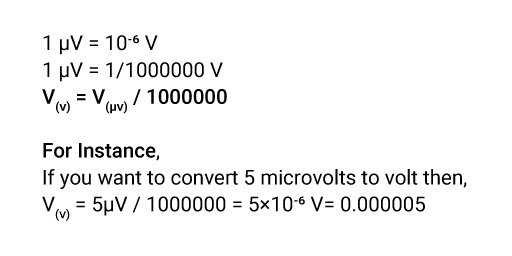## About Our µV to V Converter

“Math problems be like: Hey! I’m messed up; please fix me.”

After a long calculation, imagine ending up with a wrong outcome due to a conversion error. Who won’t get frustrated? Therefore, you can use our most trusted conversion tool to save time and get an accurate conversion answer.

Whether you want to switch microvolts to volts or another metric conversion, you are free to use our Converter.

### Definition of Microvolts.

Microvolt is the SI-derived unit of Volt that measures the Voltage. Voltage is the electrical potential difference between the 2 points of the conductors.

Microvolt is the unit of potential difference or electromotive force equal to one-millionth of a volt.

### What is the Symbol of Microvolts?

The symbol of microvolt is μV.

### How to Convert Microvolts to Volts?

One microvolt is equal to one-millionth of a volt. So, to convert the microvolts to volts, you will have to divide the Voltage by the conversion ratio. The Voltage in volts is equal to the microvolts divided by one million.

### The Microvolts to Volts Conversion Formula.

Here are the microvolts to volts conversion formula you can use to get the answer straight away.If you don’t want to play with zeros and decimals but still want an accurate answer, then you can use our microvolts to volts converter to get an answer in a flash.

### How to Use Our Microvolts to Volts Converter?

You can convert microvolts to volts easily, just like playing a game; Know-how,

• Step1: Click on our website to use our tool.
• Step2: Type the value in microvolts that you want to convert to volts.
• Step3: Press ‘convert’ to get the answer.

You can enter whichever quantity to amplify microvolts to volts. Note that click on convert every time you introduce a new value.

### How Many Microvolts Are in a Volt?

1 V = 106 µV

1V = 1000000 µV

One volt is equal to one million microvolts.

### What is the Difference Between Microvolts to Volts?

Microvolts Volts
Microvolt is a sub-multiple of Volt. Volt is the SI unit of Voltage.
µV represents the microvolts. V represents the Volt.
It is used in small quantities. Such as to simulate solar cells of different geometries. Volts are used in battery-powered portable equipment such as flashlights, cameras, etc.
1 µV = 10-6 V 1 V = 106 µV

### What is the Microvolt Unit of Measure?

Microvolt (µV) is a multiple unit of Voltage (potential difference) or electromotive force. It measures the electrical potential difference.

### Examples of Converting Microvolts to Volts.

Just go through the examples to better understand the concept of converting µV to V.

V(V) = 3µV / 1000000 = 3×10-6 V= 0.000003

V(V) = 7µV / 1000000 = 7×10-6 V= 0.000007

V(V) = 8µV / 1000000 = 8×10-6 V= 0.000008

V(V) = 10µV / 1000000 = 9×10-6 V= 0.00001

### Microvolts to Volts Conversion Table.

Here are the quick microvolts to the volts conversion table.

Microvolts Volts
1 µV 0.000001V
10 µV 0.00001V
100µV 0.0001V
1000 µV 0.001V
10000 µV 0.01V
100000 µV 0.1V
1000000 µV 1 V